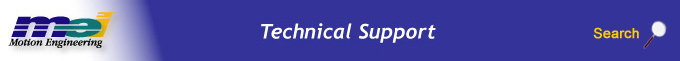.

# Comparison of Different Motion Types

## Trapezoidal

This type of motion has a trapezoidal shape for the velocity with constant acceleration and deceleration phases.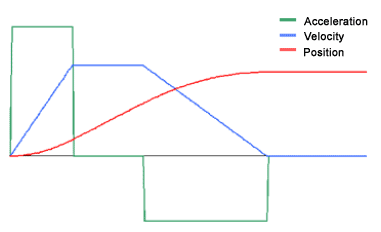## SCurve

This type of motion has an "S" shape to the acceleration and deceleration phases. It uses the JerkPercent parameter to decide how much of the acceleration and deceleration phases is curved. A JerkPercent of zero produces a trapezoidal profile.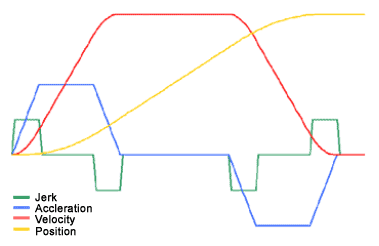## What is Jerk Percent?

Jerk percent is the percentage of time spent with non-zero jerk during the acceleration and deceleration segments of moves.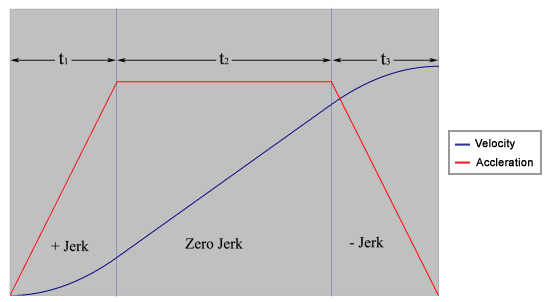For the above diagram, the jerk percent is calculated as:

 Jerk Percent = t1 + t3 t1 + t2 +t3 • 100

If the jerk percent for a move is zero, then it imitates a trapezoidal move.

For moves that use jerk percent, the acceleration and deceleration arguments represent the average acceleration and deceleration values for the move, not the peak values. As a consequence, the time of the move remains independent of the jerk percent value, which allows users to more easily calculate the time of a move. For point-to-point moves, this time is the same as a trapezoidal move with the same move parameters.

In many applications it is important to know the maximum acceleration and deceleration of a particular move. As discussed earlier, when using jerk percent, the average acceleration and decelerations are specified (not the maximum values). Fortunately, there is a simple conversion between average and maximum accelerations:

 Maximum Acceleration = average acceleration 1– (jerk percent • 0.005) • 100

## Velocity

This type of move has an "S" shape to the acceleration phase. It uses the JerkPercent argument to decide how much of the acceleration phase is curved. A JerkPercent of zero produces a trapezoidal velocity profile.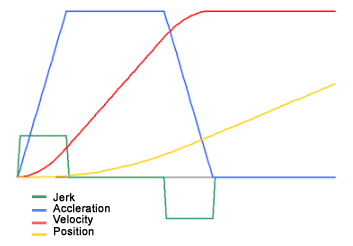## Streaming Point Motion Types

### PT Motion

PT stands for "Position - Time." A user must specify the position and time values for a given motion at multiple points.

The position for a PT move is continuous. However, the velocity is not continuous. Because of the discontinuity of velocity, PT motion should only be used when the time between points are small; otherwise spline (Bessel, Spline, BSpline, and BSpline2) or PVT motion should be used.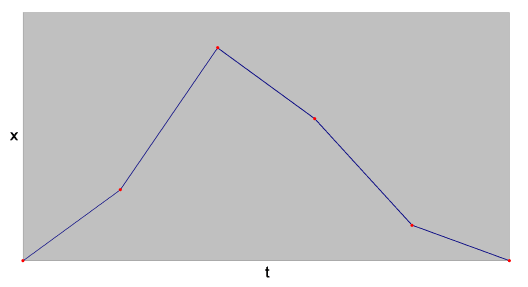PT Motion – Position vs. Time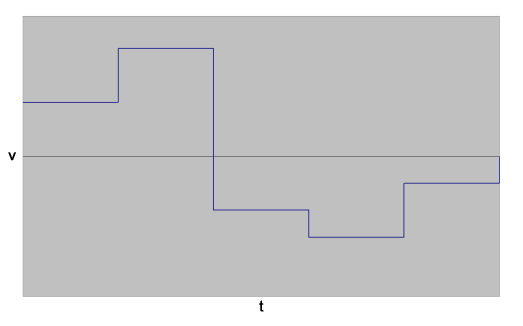PT Motion – Velocity vs. Time

### PVT Motion

PVT stands for "Position, Velocity, Time." A user must specify the position, velocity, and time values for a given motion at multiple points.

The position and velocity for a PVT move are continuous. However, the acceleration and jerk values are not continuous. The jerk maintains a constant value between PVT points, but is discontinuous at the PVT points. Therefore, the acceleration is continuous between PVT points, but it also may be discontinuous at PVT points.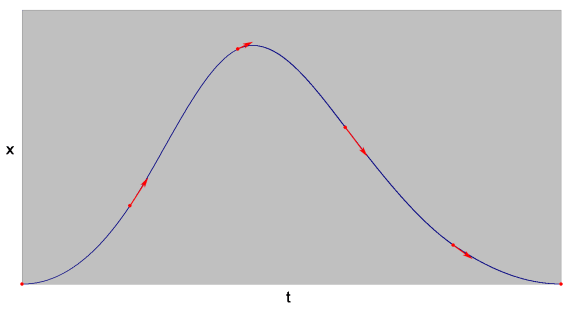PVT Motion – Position vs. Time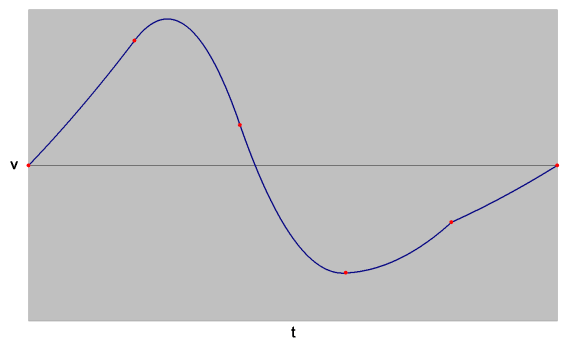PVT Motion – Velocity vs. Time

### Bessel Motion Type

With Bessel type motion, a user must specify the position and time values for a given motion at multiple points. This allows a user to create a smoother motion, but without the need to specify velocity values at each point.

For Bessel type motion, the velocity is continuous, but the acceleration is not. The velocity of Bessel type motion tends to be less smooth than the other spline motion types (Spline, B-Spline, and B-Spline2).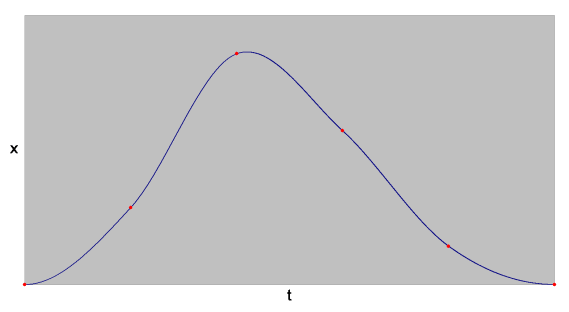Bessel Motion – Position vs. Time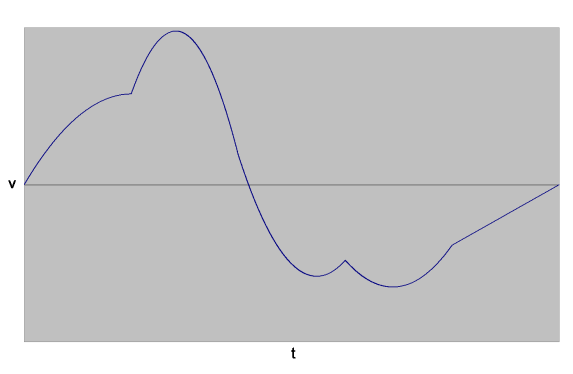Bessel Motion – Velocity vs. Time

### Spline Motion Type

With Spline type motion, a user must specify the position and time values for a given motion at multiple points. This allows a user to create a smoother motion, but without the need to specify velocity values at each point.

For Spline type motion, the velocity and acceleration are continuous. However, the accelerations at the beginning and end of a motion are not discontinuous. Also, the velocity may be discontinuous at the end of motion – however, this can be easily remedied by specifying the final point twice.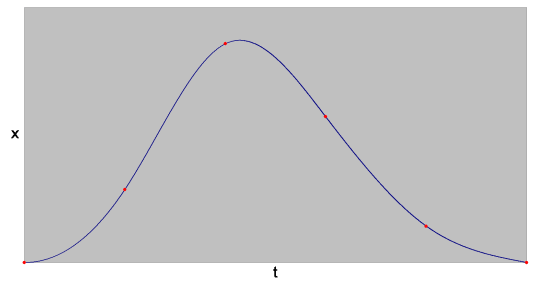Spline Motion – Position vs. Time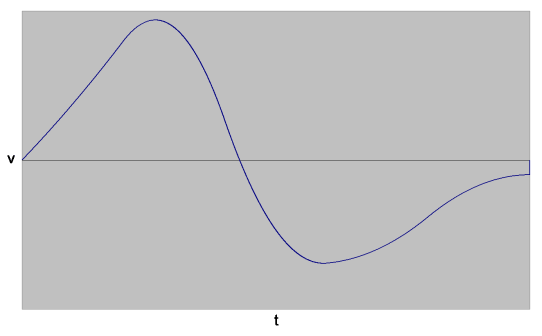Spline Motion – Velocity vs. Time

### B-Spline Motion Type

With B-Spline type motion, a user must specify the position and time values for a given motion at multiple points. This allows a user to create a smoother motion, but without the need to specify velocity values at each point.

With B-Spline motion, the generated trajectory is a 3rd order B-Spline. The velocity and acceleration are continuous. However, the accelerations at the beginning and end of motion are not discontinuous. Also, it is important to note that points specified to B-Spline motion are control points—the trajectory of the axis usually does not pass through these points; the trajectory only approaches the points.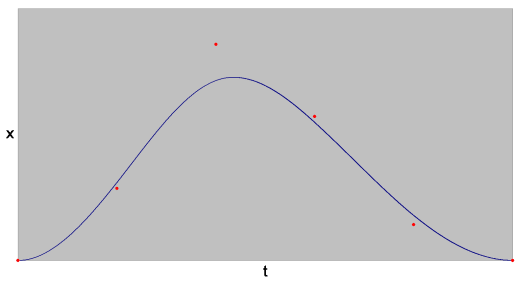B-Spline Motion – Position vs. Time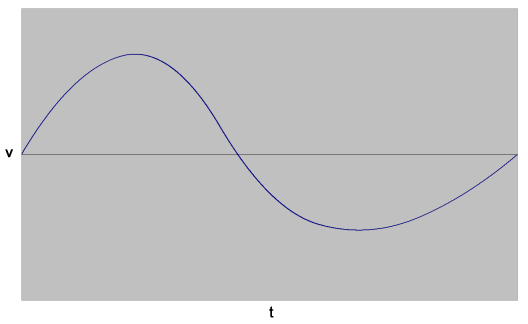B-Spline Motion – Velocity vs. Time

### B-Spline2 Motion Type

With B-Spline2 type motion, a user must specify the position and time values for a given motion at multiple points. This allows a user to create a smoother motion, but without the need to specify velocity values at each point.

With B-Spline2 motion, the generated trajectory is a 2nd order B-Spline. The velocity is continuous throughout motion, but does have a velocity jump at the start of motion. Thus it is best if the first several points in a B-Spline2 move are closely spaced in time. The acceleration is constant between control points, but discontinuous at the start and end points. Also, it is important to note that points specified to B-Spline2 motion are control points—the trajectory of the axis usually does not pass through these points; the trajectory only approaches the points.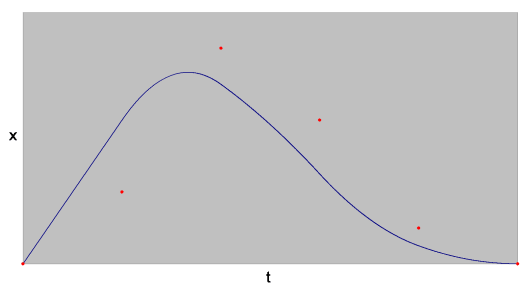B-Spline2 Motion – Position vs. Time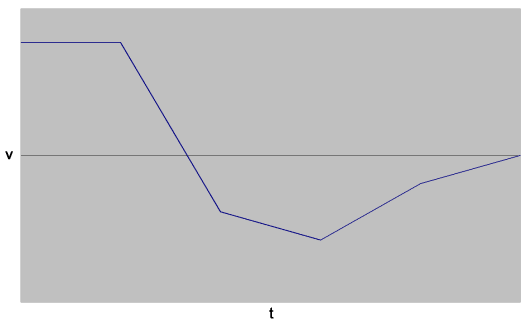B-Spline2 Motion – Velocity vs. Time

## Summary of Streaming Point Motion Types

 Motion Type Must specify entire move Segments may be appended Passes through control points PT / PTF x x PVT / PVTF x x Bessel x x x Spline x x B-Spline x x B-Spline2 x x

 Motion Type Continuous Velocity Velocity Jump at Start of Motion Velocity Jump at End of Motion Continuous Acceleration Acceleration Jump at Start of Motion Acceleration Jump at End of Motion PT / PTF x x x x PVT / PVTF† x x x Bessel x x x Spline‡ x x x x B-Spline x x x x B-Spline2 x x x † - In general, the acceleration is not continuous and acceleration jumps do occur at the beginning and end of motion for PVT moves. However, PVT moves are very flexible and it is possible to specify a move where the acceleration is continuous and no acceleration jumps occur at the beginning and end of motion. ‡ - The velocity jump at the end of a Spline move may be avoided by specifying the final point twice.

## Synchronized Motion Attributes

 Sync Start Sync End Description A new axis (labeled x' in the diagram below) is defined by the start and end point of the motion. A Cartesian coordinate system is assumed. All trajectory parameters (speed, accel, decel, ...) are used to produce motion on this axis. Only one value is needed for each trajectory parameter. x All the axes start at the same time. x All the axes stop at the same time. x x All the axes start and stop at the same time; the faster axes are slowed down to match the time of the slowest axis. Axes whose motion completes too quickly are slowed down so that all axes will complete their motion at the same time.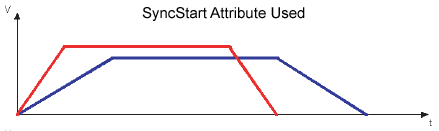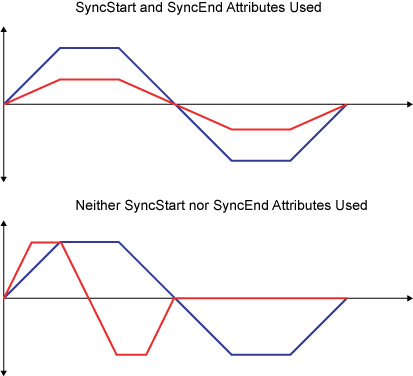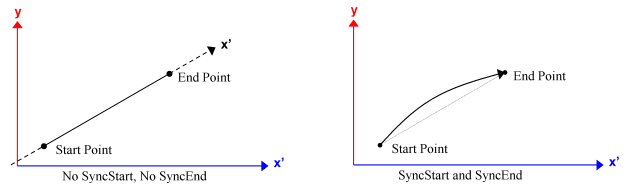Legal Notice  |  Tech Email  |  Feedback        Copyright © 2001-2010 Motion Engineering